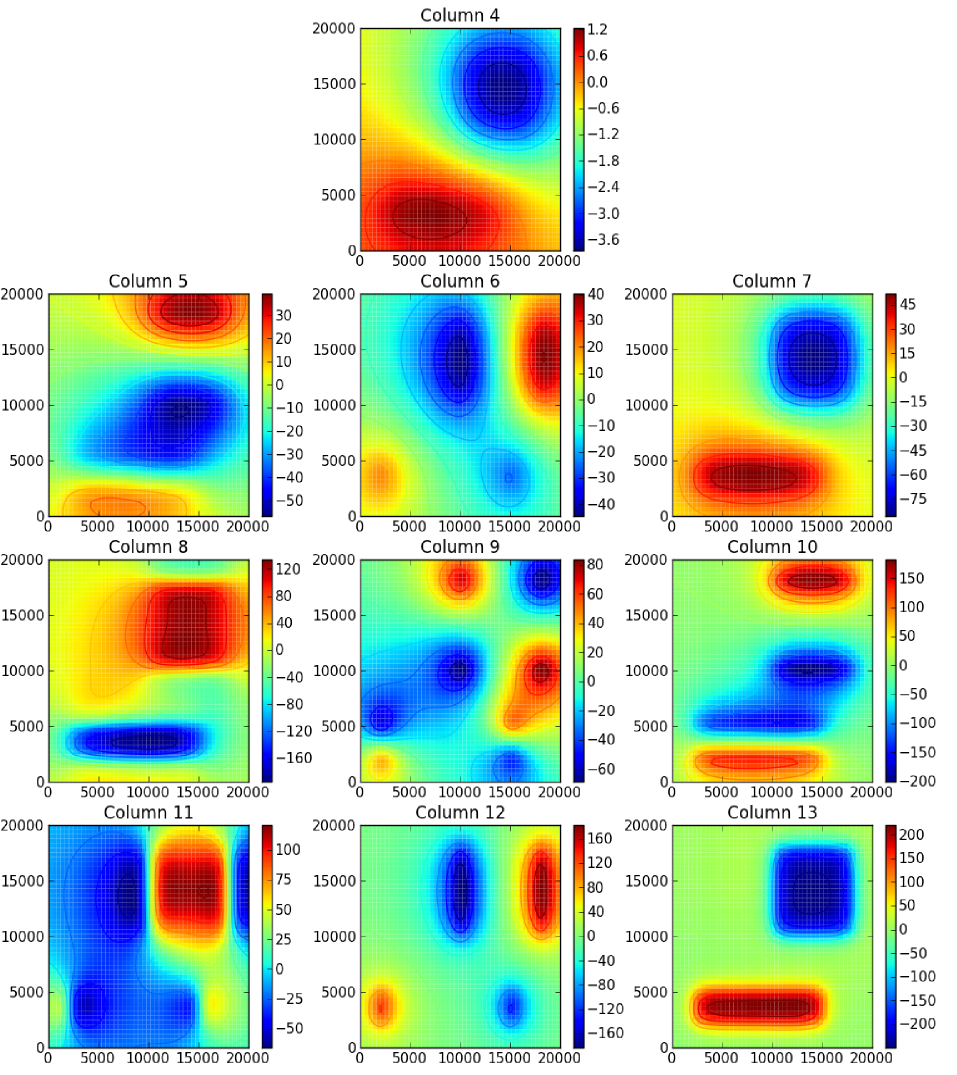# Simple prism model in Cartesian coordinates¶

The simple_prism.sh script calculates the gravitational potential, gravitational attraction, and gravity gradient tensor due to a simple prism model in Cartesian coordinates:

#!/bin/bash

# Generate a regular grid, pipe it to all the computation programs,
# and write the result to output.txt

tessgrd -r0/20000/0/20000 -b50/50 -z1000 | \
prismpot model.txt | \
prismgx model.txt | prismgy model.txt | prismgz model.txt | \
prismgxx model.txt  | prismgxy model.txt  | \
prismgxz model.txt  | prismgyy model.txt  | \
prismgyz model.txt  | prismgzz model.txt > output.txt


The model file looks like this:

# Test prism model file
2000 5000 2000 15000 0 5000 1000
10000 18000 10000 18000 0 5000 -1000


The result should look like the following (“column” means the column of the output file).Plot of the columns of output.txt generated by simple_prism.sh. The x and y axis are longitude and latitude, respectively.